# HSSlive: Plus One & Plus Two Notes & Solutions for Kerala State Board

## AP Board Class 7 Maths Chapter 7 Ratio and Proportion Review Exercise Textbook Solutions PDF: Download Andhra Pradesh Board STD 7th Maths Chapter 7 Ratio and Proportion Review Exercise Book AnswersAP Board Class 7 Maths Chapter 7 Ratio and Proportion Review Exercise Textbook Solutions PDF: Download Andhra Pradesh Board STD 7th Maths Chapter 7 Ratio and Proportion Review Exercise Book Answers

## Andhra Pradesh State Board Class 7th Maths Chapter 7 Ratio and Proportion Review Exercise Books Solutions

 Board AP Board Materials Textbook Solutions/Guide Format DOC/PDF Class 7th Subject Maths Chapters Maths Chapter 7 Ratio and Proportion Review Exercise Provider Hsslive

2. Click on the Andhra Pradesh Board Class 7th Maths Chapter 7 Ratio and Proportion Review Exercise Answers.
3. Look for your Andhra Pradesh Board STD 7th Maths Chapter 7 Ratio and Proportion Review Exercise Textbooks PDF.
4. Now download or read the Andhra Pradesh Board Class 7th Maths Chapter 7 Ratio and Proportion Review Exercise Textbook Solutions for PDF Free.

## AP Board Class 7th Maths Chapter 7 Ratio and Proportion Review Exercise Textbooks Solutions with Answer PDF Download

Find below the list of all AP Board Class 7th Maths Chapter 7 Ratio and Proportion Review Exercise Textbook Solutions for PDF’s for you to download and prepare for the upcoming exams:

Question 1.
Find the ratio of the following :
(i) 5,8
5 : 8

(ii) ₹ 10, ₹ 15
10 : 15
2 : 3
∴ ₹ 10 : ₹15 = 2 : 3

(iii) 25 kg., 20. kg.
25 kg : 20 kg
25 : 20
5 : 4
∴ 25 kg : 20 kg = 5 : 4

(iv) 5l, 500ml
5l : 500 ml
5000 ml: 500 ml (∵ 1l = 1000 ml, 5l = 5000 ml)
5000 : 500
10 : 1
∴ 5l : 500 ml = 10 : 1

(v) 2 km. 500 m, 1 km. 750 m
2 km. 500 m : 1 km. 750 m
2000 m + 500 m : 1000 m + 750 m (∵ 1 km = 1000m; 2 km = 2000 m)
2500 : 1750
10 : 7 (∵ HCF = 25)
∴ 2 km. 500 m : 1 km. 750 m = 10 : 7

(vi) 3 hrs, 1 hr. 30 min.
3 hrs : 1 hr. 30 min
3 × 60 : (1 × 60) + 30 (∵ 1 hr = 60 min; 3h = 3 × 60 = 180 min)
180 min : 60 + 30 min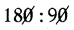2 : 1
∴ 3 hrs : 1 hr. 30 min = 2:1

(vii) 40 days, 1 year
40 days : 1 year
40 days : 365 days (∵ 1 year = 365 days)
40 : 365 (∵ HCF = 5)
8 : 73

∴ 40 days : 1 year = 8 : 73
(Or)
40 days : 366 days (∵ 1 year = 366 days for leap year)
20 : 183 (∵ HCF = 2)

Question 2.
Express the following ratios in the simplest form:
(i) 120 : 130
Given 120 : 130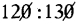12 : 13
∴ 120 : 130 = 12 : 13

(ii) 135:90
Given 135 : 90
27 : 18
3 : 2
∴ 135 : 90 = 3 : 2

(iii) 48 : 144
Given 48: 144
12 : 36
1 : 3
∴ 48 : 144 = 1:3

(iv) 81 : 54
Given 81 : 54
9 : 6
3 : 2
∴ 81 : 54 = 3 : 2

(v) 432 : 378
Given 432 : 378
216 : 189
24 : 21
8 : 7
∴ 432 : 378 = 8 : 7

Question 3.
Check whether the two ratios given below are in proportion.

(i) 10 : 20, 25 : 50
Given 10 : 20, 25 : 50
a :b = 10 : 20
So, a : b = 1 : 2

c : d = 25 : 50
also, c : d = 1 : 2

If a : b = c: d, then a, b, c, d are in proportion.
a : b = c : d = 1 : 2
Therefore, 10 : 20, 25 : 50 are in proportion.

(ii) 18 : 12, 15 : 10
Given 18 : 12, 15 : 10
a : b = 18 : 12
= 6 : 4

c : d = 15 : 10
also c : d = 3 : 2
So, a : b = 3 : 2

If a : b = c : d, then a, b, c, d are in proportion.
a : b = c : d = 3 : 2
Therefore, 18 : 12, 15 : 10 are in proportion.

(iii) 25 : 20, 16 : 14
Given 25 : 20, 16 : 14
a : b = 25 : 20
So, a : b = 5 : 4
5 : 4 ≠ 8 : 7
∴ a : b ≠ c : d

c : d = 16 : 14
c : d = 8 : 7

If a : b = c : d, then a, b, c, dare in propottion.
Here a : b ≠ c : d
So, a, b, c, d are not in proportion.

(iv) 54 : 27, 18 : 9
Given 54 : 27, 18 : 9
a : b = 54 : 27.
= 6 : 3
So, a : b = 2 : 1

c : d = 18 : 9
also, c : d = 2 : 1

If a : b = c : d, then a, b, c, d are in proportion.
a : b = c : d = 2 : 1

Therefore, 54: 27, 18 : 9 are in proportion.

Question 4.
Find the missing number in each of the following problems:
(i) 15 : 19 = 45 : _______
Given 15 : 19 = 45 : x
Let the missing term be ‘x’.
If a : b = c : d, then a, b, c and d are in proportion, then the product of extremes is equal to the product of means that is a . d = b . c
⇒ 15 × x = 19 × 45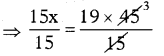⇒ x = 19 × 3
∴ x = 57

(ii) 9 : 13 = ____ : 65
Given 9 : 13 = x : 65
Let the missing term be ‘x’.
If a : b = c : d, then a, b, c and d are in proportion, then the product of extremes is equal to the product of means that is a . d = b . c
⇒ 9 × 65 = 13 × x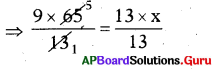⇒ 45 = x
∴ x = 45

(iii) 8 : ______ = 72 : 63
Given 8 : x = 72 : 63
Let the missing term be ‘x’.
If a : b = c : d, then a, b, c and d are in proportion, then the product of extre¬mes is equal to the product of means that is a . d = b . c
⇒ 8 × 63 = x × 72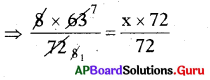⇒ 7 = x
∴ x = 7

Question 5.
Fill in the boxes with equivalent ratio of 3 : 4.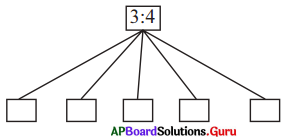Given a : b = 3 : 4 (or) 34
𝑎𝑏=34

Equivalent ratios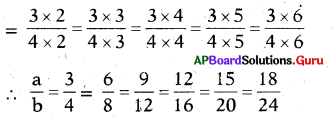Therefore, a : b = 3 : 4 = 6 : 8
= 9 : 12 = 12 : 16 = 15 : 20 = 18 : 24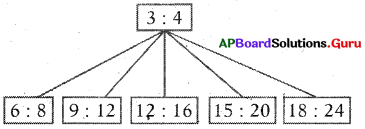Question 6.
Pick out any four numbers from below and arrange them so that they are in proportion 2, 3, 10, 12> 15, 18.
Ex : 2 : 10 = 3 : 15
(i) : __________
2 : 3; 12 : 18
a : b = 2 : 3, c : d = 12: 18
If a, b, C, d are in proportion, then the product of extremes is equal to the pro¬duct of means.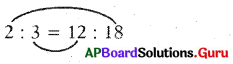a . d = b . c
2 × 18 = 3 × 12
36 = 36
So, 2 : 3 = 12 : 18

(ii) : __________
10 : 12 = 15 : 18
a : b = 10 : 12
c : d = 15 : 18
If a, b, c, d are in proportion, then the product of extremes is equal to the product of means.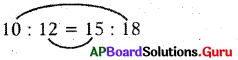a . d = b . c
10 × 18 = 15 × 12
180 = 180
So, 10 : 12 = 15 : 18

Question 7.
Divide ₹ 1500 into two parts such that they are in the ratio of 7 : 3.
Given amount to be divide = ₹ 1500 .
Ratio to be divide = 7:3
Sum of the terms in the ratio = 7 + 3 = 10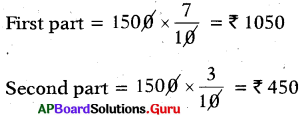[Or 1500 – 1050 = 450]

Question 8.
If there are 20 chocolates in a packet, Rajani and Ragini share them and Rajani takes 12 chocolates, then what is the ratio of chocolates taken by Rajani to Ragini?
Total number of Chocolates = 20
Number of chocolates Rajani taken = 12
Number of chocolates Ragini taken = 20 – 12 = 8
Ratio of Rajani to Ragini = 12 : 8
Ratio of chocolates taken by Rajani to Ragini = 3 : 2

Question 9.
A pipe is cut into two parts in such a ratio that the first part to second part is 7:8. If the length of the 2nd part is 48cm, then what is the length of the first part? What is the total length of the pipe before cutting?
Given ratio of two parts of pipe = 7:8
Length of the second part = 48 cm
Length of the first part = x cm
Ratio of the two parts = x : 48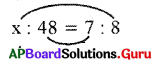Product of extremes = Product of means
⇒ 8 × x = 48 × 7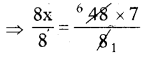⇒ x = 42
∴ Length of first part of the pipe = x = 42 cm
Length of the pipe = 42 + 48 = 90 cm

## Andhra Pradesh Board Class 7th Maths Chapter 7 Ratio and Proportion Review Exercise Textbooks for Exam Preparations

Andhra Pradesh Board Class 7th Maths Chapter 7 Ratio and Proportion Review Exercise Textbook Solutions can be of great help in your Andhra Pradesh Board Class 7th Maths Chapter 7 Ratio and Proportion Review Exercise exam preparation. The AP Board STD 7th Maths Chapter 7 Ratio and Proportion Review Exercise Textbooks study material, used with the English medium textbooks, can help you complete the entire Class 7th Maths Chapter 7 Ratio and Proportion Review Exercise Books State Board syllabus with maximum efficiency.

## FAQs Regarding Andhra Pradesh Board Class 7th Maths Chapter 7 Ratio and Proportion Review Exercise Textbook Solutions

#### Can we get a Andhra Pradesh State Board Book PDF for all Classes?

Yes you can get Andhra Pradesh Board Text Book PDF for all classes using the links provided in the above article.

## Important Terms

Andhra Pradesh Board Class 7th Maths Chapter 7 Ratio and Proportion Review Exercise, AP Board Class 7th Maths Chapter 7 Ratio and Proportion Review Exercise Textbooks, Andhra Pradesh State Board Class 7th Maths Chapter 7 Ratio and Proportion Review Exercise, Andhra Pradesh State Board Class 7th Maths Chapter 7 Ratio and Proportion Review Exercise Textbook solutions, AP Board Class 7th Maths Chapter 7 Ratio and Proportion Review Exercise Textbooks Solutions, Andhra Pradesh Board STD 7th Maths Chapter 7 Ratio and Proportion Review Exercise, AP Board STD 7th Maths Chapter 7 Ratio and Proportion Review Exercise Textbooks, Andhra Pradesh State Board STD 7th Maths Chapter 7 Ratio and Proportion Review Exercise, Andhra Pradesh State Board STD 7th Maths Chapter 7 Ratio and Proportion Review Exercise Textbook solutions, AP Board STD 7th Maths Chapter 7 Ratio and Proportion Review Exercise Textbooks Solutions,
Share: